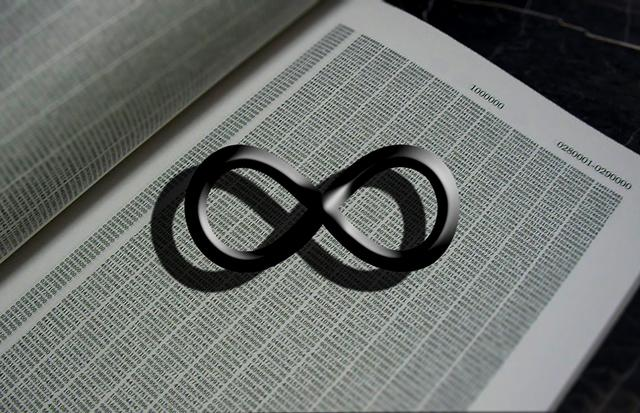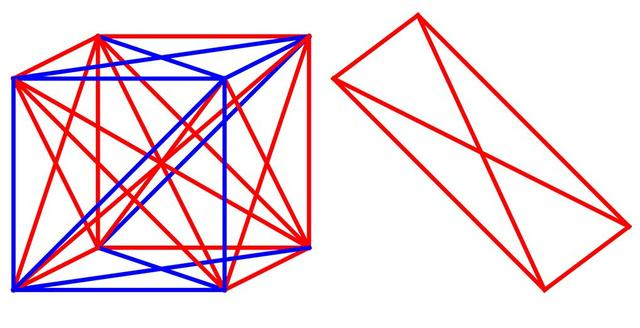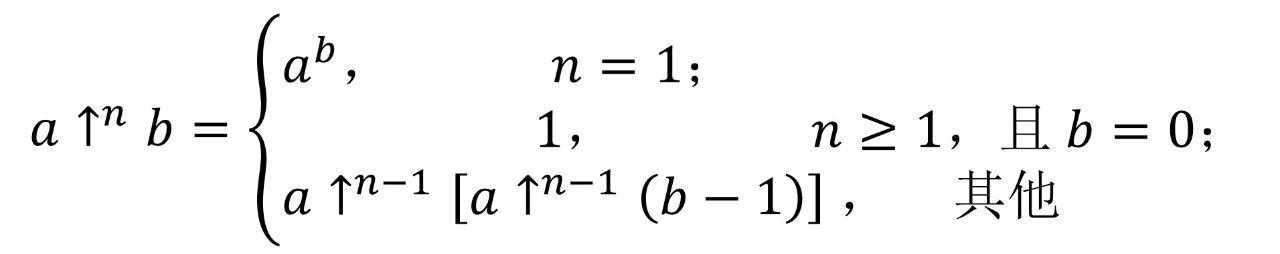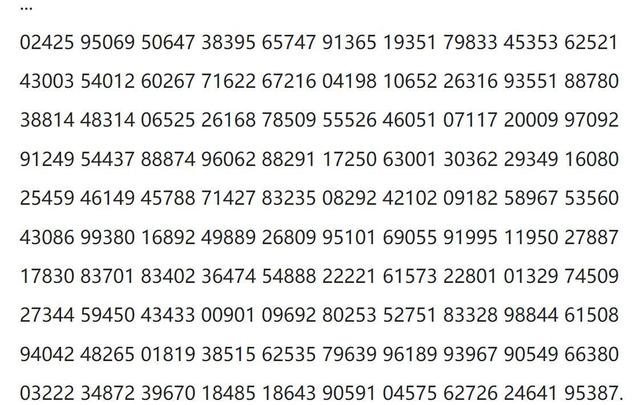0536-2756582

classify

news

0536-2756582# 葛立恒数有多大？TREE(3)又比葛立恒数大多少？3↑3=3×3×3=27

3↑↑3=3↑3↑3=3↑27=7625597484987

3↑↑↑3=3↑↑3↑↑3=3↑↑7625597484987=3^3^3^3……^3（共有3^27个3）

g(n)=3↑^g(n-1)3